Calculations Involving Percentage Solutions, Allegation and Proof Spirit : Pharmaguideline -->
 Editable Pharmaceutical Documents in MS-Word Format

# Calculations Involving Percentage Solutions, Allegation and Proof Spirit

Percent solutions, Mass percent, Calculations involving allegations, Calculations involving proof spirit.

## Calculations involving percentage solution

### Percent solutions

Among the ways to describe the concentration of a solution is by its percent solute content in a solvent. One of two methods can be used to determine the percent:

Calculating the mass ratio of the solute and the solution

This ratio is calculated by dividing the volume of the solute by the volume of the solution.

### Mass percent

The mass percent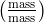is the number of grams of solute per 100 grams of the solution when the solute in a solution is solid.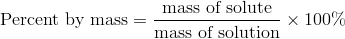Suppose 25 grams of sugar were dissolved in 100 grams of water to prepare a solution. We would calculate the mass percent by the following equation: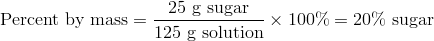A solution of a given percent by mass may require determining the mass of solvent to use sometimes when making up a specific mass of the solution. Making a 5% solution of sodium chloride, for instance, will require 3000 grams. The mass of the solute can be found by rearrangement and solving.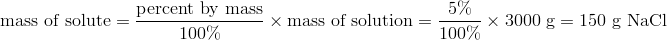Adding 150g of NaCl to 2850g of water will require you to weigh it out. For the mass of water that would need to be added, we had to subtract the mass of the NaCl (150 g) from that of the solution (3000 g).

### Calculations involving allegations

The allegation is a rapid arithmetic method used to calculate the strength of a mixture of two products. It is used to solve problems in which two products of different strengths are mixed to obtain a desired intermediate product. Alignment is derived from Latin allegation, a term which means attaching, so when allocating quantities, it refers to a line drawn during the calculation to bind quantities together.

Mixtures of two or more components of varying strengths are calculated by combining their medial alignments. Using known quantities and concentrations, it calculates the average concentration of a mixture weighing a variety of components.

Allegation calculations include the following:
• To make a desired lower strength, a given amount of preparation needs to be diluted with a given amount of diluent.
• For a given dose of lower strength preparation, the amount of active ingredient that has to be added to make a higher strength preparation.
• Combining preparations of higher and lower strengths to obtain the desired amount of intermediate strength.
Diluting a known strength preparation before compounding it as a whole is often more practical than compounding it as a whole. Alignment can sometimes be used to determine how much diluent to add to a higher strength preparation to make it the amount of strength desired.

When the strength of preparation needs to be increased, adding an active ingredient may be required. If, for example, 1% coal tar ointment is available but 2 % strength is needed, additional coal tar amounts (100%) can be added to solve the problem. By using the additive method, it is possible to determine the unknown amount.

Hence in other words we can also state that An allegation is used in the mixing of products that contain different percent strength active ingredients. In general, the final product will fall on the middle ground between each original product's strengths.

Alignment is a method that can be used to determine the required number of items of the same product, of different strengths, to achieve a final strength. Further, you can estimate the amount of each starting product you need in milliliters or grams.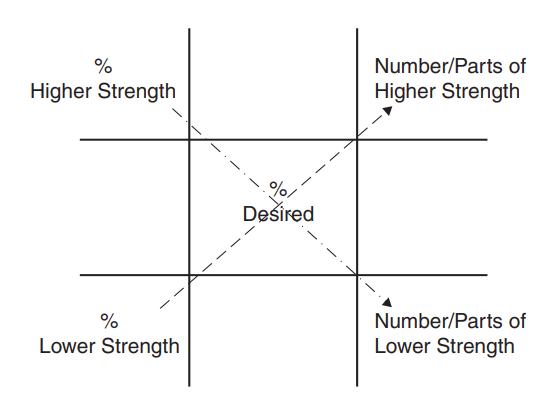## Calculations involving proof spirit

It is an excellent solvent to use alcohol. Various purposes can be served by it in pharmacy practice and cosmetic manufacturing. The concentration of alcohol in the United States Pharmacopoeia is expressed as volume-in-volume, calculated based on the amount of absolute alcohol present at 15.56 degrees Celsius. In B.P. proof spirit was officially recognized in 1885. In spiritual terms, London-proof alcohol means ethyl alcohol and water mixed at 51°F in a ratio of 12/13th of an equal volume of distilled water. Its specific gravity measure is 091976 at 15.5°C, and it contains 57.1% ethanol by volume to 100 volume of proof spirit.

The result of this is that proof spirit is a distilled liquor containing 57.1% by volume of absolute alcohol. The proof spirit of any alcohol solution containing 57.1% alcohol by volume is 100° Proof.

Overproof (°0/P) refers to alcohol with a strength greater than its proof strength.

Sub-proof alcohol (°U/P) is alcohol with a strength lower than proof.

Therefore, 25 (°U/P) means that 100 volumes of such a spirit are equivalent to 75 volumes of proof spirit. It means that 100 volumes of given alcohol correspond to 100 + 35 = 135 volumes of proof spirit. Rates of excise duty are determined by the amount of proof alcohol consumed in India.

Get subject wise printable pdf documents

Ankur Choudhary is India's first professional pharmaceutical blogger, author and founder of pharmaguideline.com, a widely-read pharmaceutical blog since 2008. Sign-up for the free email updates for your daily dose of pharmaceutical tips.

## CURRENT JOBS

 Show All ❭❭Jobs by PharmaJobs

## DOCUMENTS

PHARMACEUTICAL DOCUMENTS

Editable Pharmaceutical Documents in MS-Word Format. Ready to use SOPs, Protocols, Master Plans, Manuals and more...

View